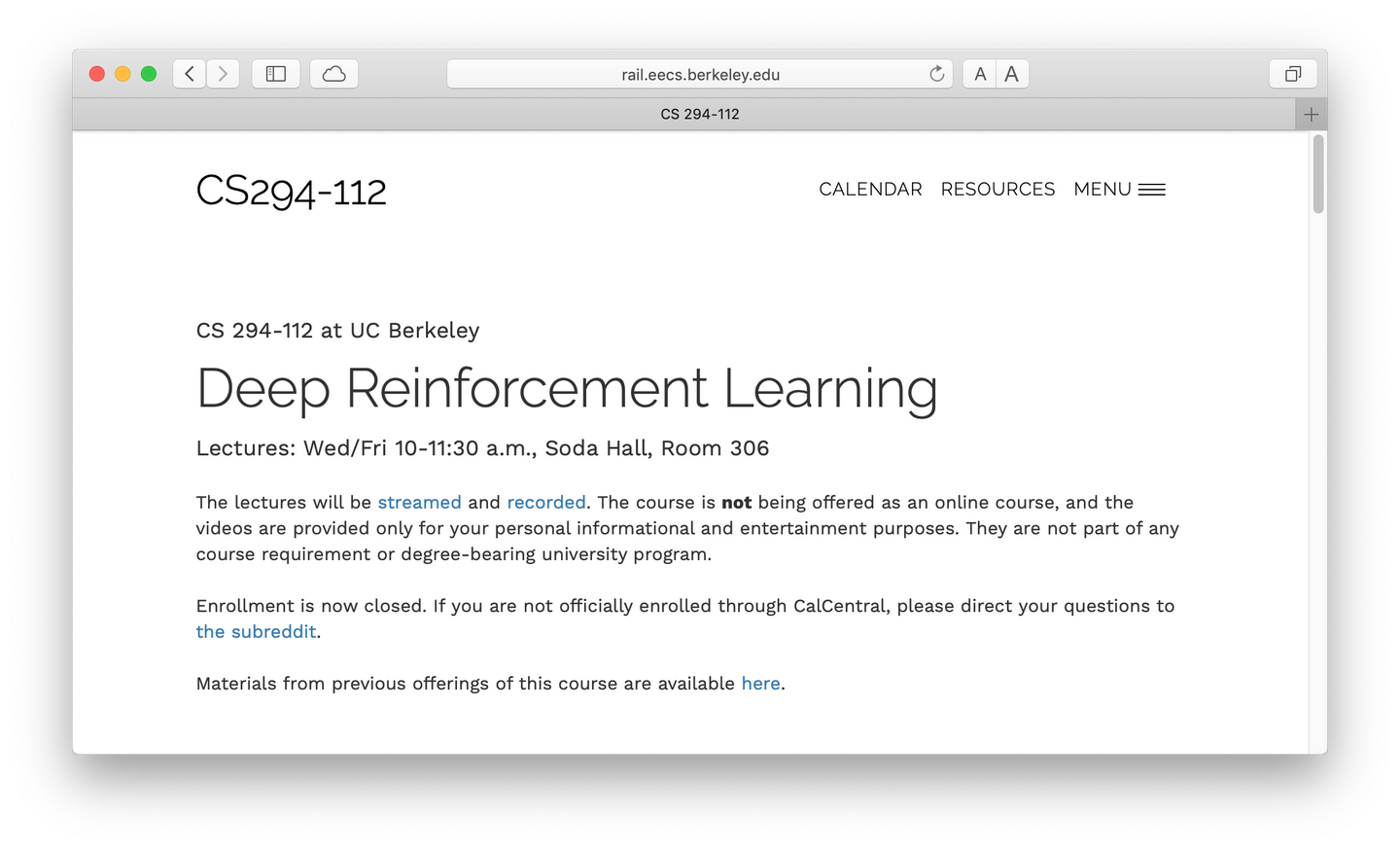目录

1. 本节任务
2. 理论背景
1. 目标是什么？
3. REINFORCE 算法
5. Value Function、Q Function 和 Advantage
6. 拟合价值函数和写出 Loss
7. 增加 Discount Factor （gamma）
8. 番外：关于连续动作空间的一些问题
3. 实验结果
4. 代码实现
5. 附录一：为什么引入常数 baseline 可以无偏地降低 policy gradient 的方差？
6. 附录二：为什么可以用 Value Function 充当 baseline？
7. 附录三：reward to go 为什么可以？

本节任务

2. 推倒 Policy Gradient 的数学形式及变形（引入 Reward to go 和 Baseline）
3. 介绍 REINFORCE 算法
4. 讨论 PG 算法的方差问题以及对策（引入 Value Function）
5. 实现 PG 算法并讨论实验结果

一、目标是什么？1. 参数是蕴含在每一时刻的策略中的
2. 策略会影响概率分布，而不是直接的影响
3.虽然具有概率分布，但是为了收获奖励，在实际环境中必须做出一个确定性的选择才行
4. 而奖励本身与参数毫无关系，奖励对参数求导为零。

PG 算法的核心任务，就是一句话：通过无偏采样的方式，得到目标函数中的数学期望，并将原来关于待解参数的不可导的目标函数变成可导的，从而可以使用 SGD 很方便的求解。三、REINFORCE 算法REINFORCE 算法的基本流程就是：

1. 执行policy，得到N个样本。
2. 计算(10)式。
3. 更新参数：4. 重复步骤1。t-1 时刻的奖励竟然会和未来的 t 时刻的动作的梯度相乘了！直观地，未来的动作不应当为之前的奖励负责。因此修改(10)式，使得 t 时刻的动作只会和 t 时刻以及之后所有的奖励发生关系，而之前的奖励与它无关。六、拟合价值函数和写出 Loss

1. 如何拟合 Value Function？
2. 能不能写出一个 Pseudo Loss，让它的梯度正好等于 Policy Gradient？这样实现起来会比较方便。七、增加 Discount Factor （gamma）1. 鼓励 agent 尽快完成任务
2. 保证了 Q 函数的收敛性，让它不会无穷大
3. 让“近期的奖励”有更大的权重，就意味着近期的数据，比如 action 和 state，对 Policy Gradient 有更大的“贡献”，因为 PG 是用蒙特卡洛采样计算出来的，如果考虑的范围越短，数据的可靠性就越高。
4. 作为 Baseline 的价值函数是拟合出来的，换言之，也是依靠蒙特卡洛采样计算出来的。如果它考虑的范围越近，拟合的精确度自然越高。Value Function(14)写成：Policy Loss：八、番外：关于连续动作空间的一些问题

1. 如果你用神经网络的输出作为动作，这个过程没有任何反馈可言，你只知道“哦，我做了这个动作，得到了这个奖励，然后呢？我该朝哪个方向改进？”
2. 在理论上没办法设计优化函数。
3. 你无法得到一个关于 action 的概率分布：代码实现

def main: 主程序，开启多个进程，没什么好讲的。
def train_PG: 单进程内跑的函数，没什么好讲的。

class Net: 基本款神经网络
class CategoricalPolicy: 离散动作空间用的策略网络
class GaussianPolicy: 连续动作空间用的策略网络

class Agent:
def sample_trajectories: 得到一组轨迹。
def sample_trajectory: 从头开始让agent和环境交互，得到一条轨迹。
def sum_of_rewards: 计算Q函数。在这里实现了reward to go。
def estimate_return: 包装了一下上面两个函数。
def update_parameters: 更新参数。

from torch import nn
from torch.multiprocessing import Process
from torch.nn import functional as F

class Net(nn.Module):
def __init__(self, obs_dim, act_dim):
super(Net, self).__init__()
self.fc0 = nn.Linear(obs_dim, 128)
self.fc1 = nn.Linear(128, act_dim)

def forward(self, x):
x = x.type_as(self.fc0.bias)
x = F.relu(self.fc0(x))
x = self.fc1(x)
return x

class GaussianPolicy(nn.Module): # 连续动作空间
def __init__(self, obs_dim, act_dim):
super(GaussianPolicy, self).__init__()
self.mean = Net(obs_dim, act_dim)
self.std = nn.Parameter(torch.ones(1, act_dim)) # 看啊，可学习变量！

def forward(self, obs):
mean = torch.tanh(self.mean(obs)) # tanh让均值归一化，惯用操作
dist = torch.distributions.Normal(mean, self.std)
action = dist.sample()
log_prob = dist.log_prob(action).sum(dim=1, keepdim=True)
return action, log_prob
# 输出log probability是标准操作，方便后续写loss

class CategoricalPolicy(nn.Module): # 离散动作空间
def __init__(self, obs_dim, act_dim):
super(CategoricalPolicy, self).__init__()
self.network = Net(obs_dim, act_dim)

def forward(self, obs):
logit = self.network(obs)
dist = torch.distributions.Categorical(logits=logit)
action = dist.sample()
log_prob = dist.log_prob(action).unsqueeze(-1)
return action, log_prob

Agent 的主体部分。

def normalize(array):  # 归一化函数，1e-7是为了避免除零。
return (array - array.mean()) / (array.std() + 1e-7)

class Agent(object):
def __init__(self, args):
...

# 定义policy network
self.policy = None
self.policy = CategoricalPolicy(self.ob_dim, self.ac_dim)
else:
self.policy = GaussianPolicy(self.ob_dim, self.ac_dim)

# 定义optimizer，看啊，PyTorch比TF方便多了。
if self.nn_baseline:
self.baseline_prediction = Net(self.ob_dim, 1)
self.baseline_loss = nn.MSELoss() # 拟合价值函数的网络的loss就是简单的L2范数

def sample_trajectories(self, itr, env):
# 采样一组轨迹，没啥好讲，调用sample_trajectory罢了。
# 高级点的玩法应当是多线程采样，不过这个以后再说。
...
while 采样数目不到的时候:
ob, ac, log_prob, re = self.sample_trajectory(env, animate_this_episode)
... # 包装数据
return np.concatenate(obs), acs, torch.cat(log_probs), res, timesteps_this_batch

def sample_trajectory(self, env, animate_this_episode):
# 采样一条轨迹。实际上就是类似于Homework1中的test函数。
ob = env.reset()
obs, acs, rewards, log_probs = [], [], [], []
for steps in count(): # 一个语法糖，用itertools中的count类，等价于死循环和一个自加变量。
ac, log_prob, _ = self.policy(torch.from_numpy(ob).to(self.device).unsqueeze(0))
if self.discrete: # PyTorch不同数据格式的转换是有点麻烦的
ac = ac.item()
else:
ac = ac.cpu().detach().numpy()
obs.append(ob)
log_probs.append(log_prob.squeeze(-1))
acs.append(ac)
ob, rew, done, _ = env.step(ac) # 与环境互动，传入的是Numpy数组
rewards.append(rew)
if done or steps > self.max_path_length:
break
return np.array(obs), np.array(acs), torch.cat(log_probs), np.array(rewards),

def sum_of_rewards(self, re_n):
# 上面都是一些事务性的代码。现在进入正题。
q_n = []
for tau in re_n:
result = []
Q = 0
for r in tau[::-1]: # 这里是利用Q函数的迭代性计算Q。
Q = r + self.gamma * Q # 等于式子(20)
result.insert(0, Q) # 如果采用Reward to go，就要倒序地向result中插入Q。
if not self.reward_to_go: # 如果不采用，就用一条轨迹的总奖励，复制若干次作为结果。
result = [Q for _ in range(len(tau))]
q_n.extend(result) # list的extend函数。也可以写成list += [1,2,3]
return np.array(q_n)

q_n = torch.from_numpy(q_n).to(self.device) # 从numpy数组变成tensor
if self.nn_baseline:
# 调用价值函数网络，得到预测值。里面一大串是numpy数组转换成tensor。
b_n = self.baseline_prediction(torch.from_numpy(ob_no).to(self.device))
# 归一化网络的输出，再让它与这个batch的Q值的分布一样。
b_n = normalize(b_n) * q_n.std() + q_n.mean()  # match the statistics of Q values
else:

def estimate_return(self, ob_no, re_n): # 调用了上面两个函数
q_n = self.sum_of_rewards(re_n)

def update_parameters(self, ob_no, log_prob_na, q_n, adv_n):
# 看啊，PyTorch比TF方便了多少，简单几句话就写完了训练过程。
if self.nn_baseline:
# 得到价值函数拟合网络的输出
prediction = self.baseline_prediction(torch.from_numpy(ob_no).to(self.device).unsqueeze(0))
# 目标函数就是Q函数。并且进行了归一化。（回忆一下上面，让网络输出归一化到Q的分布）
target = normalize(torch.from_numpy(q_n).to(self.device)).type_as(prediction)
loss = self.baseline_loss(input=prediction, target=target) # 平方误差
loss.backward()
self.baseline_optimizer.step()

loss.backward()
self.optimizer.step()

附录一：为什么引入常数 baseline 可以无偏地降低 policy gradient 的方差？

1. 它的引入会不会导致式子(10)对式子(9)的估计失效了，即它的引入是否伤害无偏性？
2. 它的引入能不能降低方差？附录二：为什么可以用 Value Function 充当 baseline？附录三：Reward to go 为什么可以？